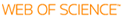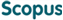BROWSE

Related ResearcherJang, Bongsoo
Computational Mathematical Science Lab (CMS Lab)
Research Interests
• Numerical analysis, dynamics, fractional systems, data sciences

Comments on "Solving a class of two-dimensional linear and nonlinear Volterra integral equations by the differential transform method"

Cited 6 times inCited 8 times inTitle
Comments on "Solving a class of two-dimensional linear and nonlinear Volterra integral equations by the differential transform method"
Author
Issue Date
2009-11
Publisher
ELSEVIER SCIENCE BV
Citation
JOURNAL OF COMPUTATIONAL AND APPLIED MATHEMATICS, v.233, no.2, pp.224 - 230
Abstract
Tari et al. [A. Tari, M.Y. Rahimi, S. Shahmorad, F. Talati, Solving a class of two-dimensional linear and nonlinear Volterra integral equations by the differential transform method, J. Comput. Appl. Math. 228 (2009) 70-76], presented some fundamental properties of TDTM for the kernel functions in two-dimensional Volterra integral equations. Here, we suggest simple proofs of those fundamental properties by using the basic properties of TDTM. Furthermore, we present some fundamental properties of TDTM for the kernel functions of a quotient type in two-dimensional Volterra integral equations. Numerical illustrations are demonstrated to show the effectiveness of the TDTM for solving two-dimensional Volterra integral equations.
URI
https://scholarworks.unist.ac.kr/handle/201301/3150
URL
http://www.scopus.com/inward/record.url?partnerID=HzOxMe3b&scp=69249229467
DOI
10.1016/j.cam.2009.07.012
ISSN
0377-0427
Appears in Collections:
MTH_Journal Papers
Files in This Item:
There are no files associated with this item.